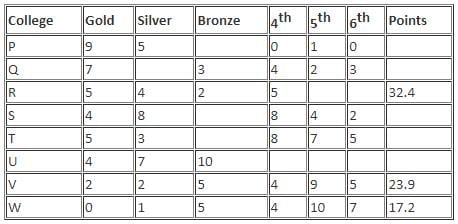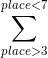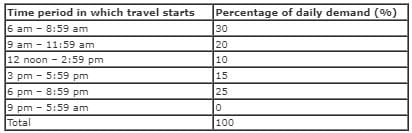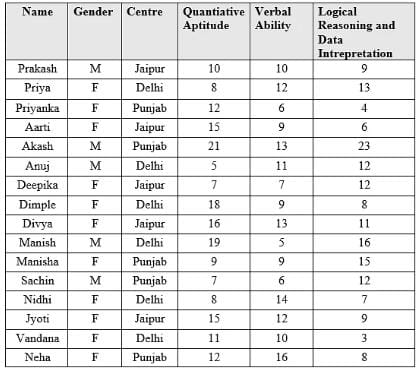CAT  >  Practice Questions Level 3: Caselets - 1

# Practice Questions Level 3: Caselets - 1 - Notes | Study Level-wise Practice Questions for CAT Preparation - CAT

 1 Crore+ students have signed up on EduRev. Have you?

This EduRev document offers 10 Multiple Choice Questions (MCQs) from the topic Caselets (Level - 3). These questions are of Level - 3 difficulty and will assist you in the preparation of CAT & other MBA exams. You can practice/attempt these CAT Multiple Choice Questions (MCQs) and check the explanations for a better understanding of the topic.

Question for Practice Questions Level 3: Caselets - 1
Try yourself:Directions: Refer to the data below and answer the question that follows.

The table below gives the number of times 8 colleges viz. P, Q, R, S, T, U, V and W won gold, silver or bronze medals and obtained 4th, 5th and 6th places in intercollege competitions held this year.The number of points the college scored during the competitions is calculated using the following formula:
Number of points = (Gold medals) × 3 + (Silver medals) × 2 + (Bronze medals) +[(1 - 0.1 × place) × (places)
[Note: (places) or (medals) refers to the number of times the college won that place or that medal]
Some additional facts that are known are as follows:
(i) The number of bronze medals won by college P is the same as the number of silver medals won by college Q.
(ii) The number of times college U was at the 4th place is the same as the number of bronze medals won by college T.
(iii) No two colleges are assigned the same place in a game.

Q. How many times did college P win the bronze medal?

Question for Practice Questions Level 3: Caselets - 1
Try yourself:Directions: Refer to the data below and answer the question that follows.

The table below gives the number of times 8 colleges viz. P, Q, R, S, T, U, V and W won gold, silver or bronze medals and obtained 4th, 5th and 6th places in intercollege competitions held this year.The number of points the college scored during the competitions is calculated using the following formula:
Number of points = (Gold medals) × 3 + (Silver medals) × 2 + (Bronze medals) +[(1 - 0.1 × place) × (places)
[Note: (places) or (medals) refers to the number of times the college won that place or that medal]
Some additional facts that are known are as follows:
(i) The number of bronze medals won by college P is the same as the number of silver medals won by college Q.
(ii) The number of times college U was at the 4th place is the same as the number of bronze medals won by college T.
(iii) No two colleges are assigned the same place in a game.

Q. How many colleges scored more than 40 points?

Question for Practice Questions Level 3: Caselets - 1
Try yourself:Directions: Refer to the data below and answer the question that follows:
The daily demand (in number of customers) for air travel from Delhi to Bangalore and from Bangalore to Delhi, each is 1200 customers. The breakdown of demand by time is given in the following table:The demand for travel from Bangalore to Delhi is identical to that from Delhi to Bangalore. Any unmet demand in any time period does not spill over to the next time period.
Space jet, an airline, which flies only between Delhi and Bangalore has the following costs:
1. A surcharge A per passenger of Rs. 100 to be paid to the Airlines Regulatory Board.
2. A fixed cost B of Rs. 72000 per trip made.
The airline owns 2 planes, each with a maximum capacity of 150 passengers. One plane is parked at Delhi and the other at Bangalore at the beginning of the day. One trip (from Delhi to Bangalore or vice versa) takes 1.5 hours. The airline charges a uniform fare of Rs. 1000 per passenger per trip.

Customer satisfaction index (CSI) is defined as:

CSI = 1 - (Unmet demand)/(Total number of passengers that the airline carries on that day)

Q. Let P be the maximum profit possible in the first time period of 6 am to 8:59 am. Based on new information, it is known that 60% of the demand in the first time period consists of business travellers who will pay Rs. 2500 per trip, but will not wait beyond 7 am. Hence, the airline company decided to purchase two more planes with a capacity of 150 each. If it has to maintain the same profit P, what is the fixed cost per trip that the airline has to pay for the new plane? Assume the same surcharge of Rs. 100 per passenger.

Question for Practice Questions Level 3: Caselets - 1
Try yourself:Directions: Refer to the data below and answer the question that follows:
The daily demand (in number of customers) for air travel from Delhi to Bangalore and from Bangalore to Delhi, each is 1200 customers. The breakdown of demand by time is given in the following table:The demand for travel from Bangalore to Delhi is identical to that from Delhi to Bangalore. Any unmet demand in any time period does not spill over to the next time period.
Space jet, an airline, which flies only between Delhi and Bangalore has the following costs:
1. A surcharge A per passenger of Rs. 100 to be paid to the Airlines Regulatory Board.
2. A fixed cost B of Rs. 72000 per trip made.
The airline owns 2 planes, each with a maximum capacity of 150 passengers. One plane is parked at Delhi and the other at Bangalore at the beginning of the day. One trip (from Delhi to Bangalore or vice versa) takes 1.5 hours. The airline charges a uniform fare of Rs. 1000 per passenger per trip.

Customer satisfaction index (CSI) is defined as:

CSI = 1 - (Unmet demand)/(Total number of passengers that the airline carries on that day)

Q. If the airline wants to make exactly 10 trips (5 each way) per day in such a way that maximises the profit for the airline, which of the following cannot be the start time for the third flight from Delhi to Bangalore?

Question for Practice Questions Level 3: Caselets - 1
Try yourself:Directions: Answer the question on the basis of the information given below.
The following table provides information about the marks obtained by following sixteen students in Quantitative Aptitude, Logical Reasoning and Data Interpretation and Verbal Ability in an MBA entrance test for an MBA Institute.
(i) The total marks obtained by the student in the test is the sum of the marks obtained by the student in the mentioned three sections. It also provides information about the centre at which the student is enroled.
(ii) The students belong to either one of the three centres namely Jaipur, Delhi and Punjab. Each student is enroled at only one centre.
(iii) Each student is given only one rank from 1 to 16 based on the marks obtained by him/her in the entrance test. This rank is called 'overall rank'.
Note:
A student A (assume) is given a numerically lesser rank than the other student B (assume) if the total marks obtained by A is greater than the total marks obtained by B.
• If the total marks obtained by A is same as those obtained by B, then the student having obtained more marks in Verbal Ability section is given a numerically lesser rank.
• If marks obtained by two students in Verbal Ability section are also same, then the student having obtained more marks in Logical Reasoning and Data Interpretation section is given a numerically lesser rank.Q. From which of the following centres did the maximum possible number of students obtain a total of at least 33 marks and a total of at most 57 marks?

Question for Practice Questions Level 3: Caselets - 1
Try yourself:Directions: Answer the question on the basis of the information given below.
The following table provides information about the marks obtained by following sixteen students in Quantitative Aptitude, Logical Reasoning and Data Interpretation and Verbal Ability in an MBA entrance test for an MBA Institute.
(i) The total marks obtained by the student in the test is the sum of the marks obtained by the student in the mentioned three sections. It also provides information about the centre at which the student is enroled.
(ii) The students belong to either one of the three centres namely Jaipur, Delhi and Punjab. Each student is enroled at only one centre.
(iii) Each student is given only one rank from 1 to 16 based on the marks obtained by him/her in the entrance test. This rank is called 'overall rank'.
Note:
A student A (assume) is given a numerically lesser rank than the other student B (assume) if the total marks obtained by A is greater than the total marks obtained by B.
• If the total marks obtained by A is same as those obtained by B, then the student having obtained more marks in Verbal Ability section is given a numerically lesser rank.
• If marks obtained by two students in Verbal Ability section are also same, then the student having obtained more marks in Logical Reasoning and Data Interpretation section is given a numerically lesser rank.Q. How many male student(s) has/have obtained more marks in Logical Reasoning and Data Interpretation than the marks obtained by Deepika in Logical Reasoning and Data Interpretation, but less marks in Verbal Ability than the marks obtained by Dimple in Verbal Ability?

Question for Practice Questions Level 3: Caselets - 1
Try yourself:Directions: Answer the following question based on the information given below.
Tina, a blast furnace expert, who works as a technology trouble shooter stays in Jamshedpur. She has got an important assignment in Delhi, which requires 6 hours to complete. The work is so critical that she has to start working the moment she reaches the client's premises.
She is considering various options for her onward and return journey between Jamshedpur and Delhi.
A quick search revealed that ticket from Jamshedpur to Delhi is available on 2 trains. Trains 12801 and 12443 depart from Jamshedpur station at 06:45 hrs and 15:55 hrs and reach Delhi next day at 04:50 hrs and 10:35 hrs, respectively. Trains 12444 and 12802 start from Delhi at 17:20 hrs and 22:20 hrs and reach Jamshedpur next day at 10:35 hrs and 20:05 hrs, respectively.
Another option is to reach Ranchi by a 3-hour road trip and take a flight to Delhi from Ranchi. The distance between Delhi and Ranchi is covered in 105 minutes both ways by any of the scheduled flights. Air India operates 2 flights AI 9810 and AI 810, which depart Ranchi at 08:00 hours and 15:25 hrs, respectively. Flight number IT - 3348 operated by Kingfisher Airlines departs Ranchi at 19:20 hrs. Return flights operated by Air India - AI 9809 and AI809 depart Delhi at 05:50 hrs and 11:00 hrs, respectively. Flight number IT - 3347 operated by Kingfisher Airlines departs Delhi at 17:10 hrs.
From Tina's home, Jamshedpur railway station is 5 minutes drive, and her destination at Delhi is 90 minutes and 30 minutes drive from airport and railway station, respectively. One has to reach the airport at least 1 hour before the scheduled departure to complete the boarding procedure. At every railway station, she loses 5 minutes in navigating through the crowd.

Q. If Tina decides to minimise the in-between waiting period, the option she should choose from the ones given below will be:

Question for Practice Questions Level 3: Caselets - 1
Try yourself:Directions: Answer the following question based on the information given below.
Tina, a blast furnace expert, who works as a technology trouble shooter stays in Jamshedpur. She has got an important assignment in Delhi, which requires 6 hours to complete. The work is so critical that she has to start working the moment she reaches the client's premises.
She is considering various options for her onward and return journey between Jamshedpur and Delhi.
A quick search revealed that ticket from Jamshedpur to Delhi is available on 2 trains. Trains 12801 and 12443 depart from Jamshedpur station at 06:45 hrs and 15:55 hrs and reach Delhi next day at 04:50 hrs and 10:35 hrs, respectively. Trains 12444 and 12802 start from Delhi at 17:20 hrs and 22:20 hrs and reach Jamshedpur next day at 10:35 hrs and 20:05 hrs, respectively.
Another option is to reach Ranchi by a 3-hour road trip and take a flight to Delhi from Ranchi. The distance between Delhi and Ranchi is covered in 105 minutes both ways by any of the scheduled flights. Air India operates 2 flights AI 9810 and AI 810, which depart Ranchi at 08:00 hours and 15:25 hrs, respectively. Flight number IT - 3348 operated by Kingfisher Airlines departs Ranchi at 19:20 hrs. Return flights operated by Air India - AI 9809 and AI809 depart Delhi at 05:50 hrs and 11:00 hrs, respectively. Flight number IT - 3347 operated by Kingfisher Airlines departs Delhi at 17:10 hrs.
From Tina's home, Jamshedpur railway station is 5 minutes drive, and her destination at Delhi is 90 minutes and 30 minutes drive from airport and railway station, respectively. One has to reach the airport at least 1 hour before the scheduled departure to complete the boarding procedure. At every railway station, she loses 5 minutes in navigating through the crowd.

Q. Tina gets a message that her work has to be completed between 09:00 hrs and 17:00 hrs. If she wants to minimise the total time out of Jasmshedpur, the best option, from among the following, is to go by:

Question for Practice Questions Level 3: Caselets - 1
Try yourself:Directions: Read the information carefully to answer the question that follows.
Dr. Ravi Patel teaches financial markets course at Indian Management Institute, which is being taught in two parts - one part in first trimester and the other part in second trimester. Initially, the professor divides the students randomly in five classes in first trimester and based on the performance of students in first trimester, he further divides the students of each class into two parts as below average and above average performers. It is observed that in each class, the ratio of below average and above average performers is at least 13/10.
Initially, the classes were named as classes P, Q, R, S and T and after further division, the classes were renamed as classes 1, 2, 3, 4 ,....,10 and the classes in trimester 2 are named randomly i.e. it is not necessary that two parts of class A are numbered one after the other.
It is also given that 1/5 of total students in first trimester were in class R, 23% of total students were in class Q and 17% of total students were in class T. The ratio of students in classes P and S was 11 : 9. In the second trimester, there were 20 students in class 1. The number of students in classes 3, 10 and 9 were twice, thrice and four times the number of students in class 1. The number of students in classes 4 and 5 are in ratio 1 : 3. The number of students in class 2 were 5 more than the number of students in class 9. The number of students in class 8 was 40% more than the number of students in class 4. The number of students in classes 6 and 7 is 50 and 30 and the total number of students in classes 4, 5 and 8 is 125% more than the number of students in class 10. Each student in first trimester was classified as either below average or above average performer.

Q. In how many classes was the number of students who performed below average at least 90% more than the number of students who performed above average?

Question for Practice Questions Level 3: Caselets - 1
Try yourself:Directions: Read the information carefully to answer the question that follows.
Dr. Ravi Patel teaches financial markets course at Indian Management Institute, which is being taught in two parts - one part in first trimester and the other part in second trimester. Initially, the professor divides the students randomly in five classes in first trimester and based on the performance of students in first trimester, he further divides the students of each class into two parts as below average and above average performers. It is observed that in each class, the ratio of below average and above average performers is at least 13/10.
Initially, the classes were named as classes P, Q, R, S and T and after further division, the classes were renamed as classes 1, 2, 3, 4 ,....,10 and the classes in trimester 2 are named randomly i.e. it is not necessary that two parts of class A are numbered one after the other.
It is also given that 1/5 of total students in first trimester were in class R, 23% of total students were in class Q and 17% of total students were in class T. The ratio of students in classes P and S was 11 : 9. In the second trimester, there were 20 students in class 1. The number of students in classes 3, 10 and 9 were twice, thrice and four times the number of students in class 1. The number of students in classes 4 and 5 are in ratio 1 : 3. The number of students in class 2 were 5 more than the number of students in class 9. The number of students in class 8 was 40% more than the number of students in class 4. The number of students in classes 6 and 7 is 50 and 30 and the total number of students in classes 4, 5 and 8 is 125% more than the number of students in class 10. Each student in first trimester was classified as either below average or above average performer.

Q. Out of total students in class S, what percent performed below average?

The document Practice Questions Level 3: Caselets - 1 - Notes | Study Level-wise Practice Questions for CAT Preparation - CAT is a part of the CAT Course Level-wise Practice Questions for CAT Preparation.
All you need of CAT at this link: CAT

## Level-wise Practice Questions for CAT Preparation

277 docs
 Use Code STAYHOME200 and get INR 200 additional OFF

## Level-wise Practice Questions for CAT Preparation

277 docs

### How to Prepare for CAT

Read our guide to prepare for CAT which is created by Toppers & the best Teachers

Track your progress, build streaks, highlight & save important lessons and more!

,

,

,

,

,

,

,

,

,

,

,

,

,

,

,

,

,

,

,

,

,

;